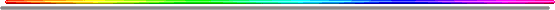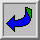If A = 1, B = 2, C = 3...

- Jerusalem = 104

- The measure of the celestial Jerusalem is of 144 cubits, both an angel's measure and a man's measure.

" [... ] And he measured the wall thereof, an hundred and forty and four cubits, according to the measure of a man, that is, of the angel. ". (Revel. 21.17). -

- Every (not bissextile) year, the 104th day falls on April 14, or the 14.4. (if written according to the French datation system).

- 104' = 1.44' (because 104 minutes... are also 1h.44).

- A day counts 60 minutes x 24 hours or 1440 minutes. (See the symbolism of the 24 old men in the Revelation).

As a consequence, 100 days can be converted into 144 000 minutes and the 100th day falls on the 10.4, (if written according to the French datation system and if the considered year is not bissextile).

- every 108th month, the 10.4 date is also a [ 1-4-4 ] combination, (if written according to the French datation system and if the considered year is not bissextile)..

- A date like 10.4 can end on a Saturday, at the 144th hour of the week such as (Saturday) the 10.4.1993 which was also a [1-4-4] combination (as far as 1 + 9 + 9 + 3 = 22 = 2 + 2 = 4). (if written according to the French datation system).

or

- begin on a Sunday after the 144th hour of the week such as the 10.4.1994 or even better, the 10.4.1966, another [1-4-4] combination which is even recorded itself in the date.

A French word such " jour (day) " written in the singular, appears 1441 times in the whole French (Lsg) Bible and the French words " heure(s) - (hour(s))", " vision(s) - (vision(s)) "... 104 times.

The 144th verse as follows:

" [... ] But the seventh day is the Sabbath of the LORD thy God: in it thou shalt not do any work, thou, nor thy son, nor thy daughter, thy manservant, nor thy maidservant, nor thy cattle, nor thy stranger that is within thy gates:". (Ex 20/10).

The 7th day begins right after the 144-th hour of a week.

- 104 instruments are necessary to carry out sacrifice rites in the (actually destroyed) temple of Jerusalem. (This set has been recently completely reconstituted by those who prepare in Jerusalem the reconstruction of the Temple and the revival of the ritual rites during the cult)...

Table of the first hundred multiples of number 104:

 104 x 1 = 104 104 x 35 = 3640 104 x 69 = 7176 104 x 2 = 208 104 x 36 = 3744 104 x 70 = 7280 104 x 3 = 312 104 x 37 = 3848 104 x 71 = 7384 104 x 4 = 416 104 x 38 = 3952 104 x 72 = 7488 104 x 5 = 520 104 x 39 = 4056 104 x 73 = 7592 104 x 6 = 624 104 x 40 = 4160 104 x 74 = 7696 104 x 7 = 728 104 x 41 = 4264 104 x 75 = 7800 104 x 8 = 832 104 x 42 = 4368 104 x 76 = 7904 104 x 9 = 936 104 x 43 = 4472 104 x 77 = 8008 104 x 10 = 1040 104 x 44 = 4576 104 x 78 = 8112 104 x 11 = 1144 104 x 45 = 4680 104 x 79 = 8216 104 x 12 = 1248 104 x 46 = 4784 104 x 80 = 8320 104 x 13 = 1352 104 x 47 = 4888 104 x 81 = 8424 104 x 14 = 1456 104 x 48 = 4992 104 x 82 = 8528 104 x 15 = 1560 104 x 49 = 5096 104 x 83 = 8632 104 x 16 = 1664 104 x 50 = 5200 104 x 84 = 8736 104 x 17 = 1768 104 x 51 = 5304 104 x 85 = 8840 104 x 18 = 1872 104 x 52 = 5408 104 x 86 = 8944 104 x 19 = 1976 104 x 53 = 5512 104 x 87 = 1218 104 x 20 = 2080 104 x 54 = 5616 104 x 88 = 9152 104 x 21 = 2184 104 x 55 = 5720 104 x 89 = 9256 104 x 22 = 2288 104 x 56 = 5824 104 x 90 = 9360 104 x 23 = 2392 104 x 57 = 5928 104 x 91 = 9464 104 x 24 = 2496 104 x 58 = 6032 104 x 92 = 9568 104 x 25 = 2600 104 x 59 = 6136 104 x 93 = 9672 104 x 26 = 2704 104 x 60 = 6240 104 x 94 = 9776 104 x 27 = 2808 104 x 61 = 6344 104 x 95 = 9880 104 x 28 = 2912 104 x 62 = 6448 104 x 96 = 9984 104 x 29 = 3016 104 x 63 = 6552 104 x 97 = 10088 104 x 30 = 3120 104 x 64 = 6656 104 x 98 = 10192 104 x 31 = 3224 104 x 65 = 6760 104 x 99 = 10296 104 x 32 = 3328 104 x 66 = 6864 104 x 100 = 10400 104 x 33 = 3432 104 x 67 = 6968 ... 104 x 34 = 3536 104 x 68 = 7072Bible and Numbers End Times Interpreted with the Help of the Bible, Numbers, and the French Language, by Anne E. Crews and Gérard Colombat (author of this website) "Was 9/11 supposed to happen, and is it related to the Rapture of the Christian Church? If so, how? Are all world events based on a coded numerical system, and if so, to whom does the coded numerical system point…the MESSIAH Jesus Christ? Bible and Numbers is a factual attempt to answer these lingering questions. Based on over thirty years of research by author Gérard Colombat, authors Anne E. Crews and Colombat proffer an intelligent and insightful theory in their quest for biblical truth. By juxtaposing a numerical system based on the modern calendar, sacred Bible numbers, and the phonetics and numerics of the French language, Colombat and Crews seek to define the occurrences of past events in conjunction with the present, offering that through the Bible and Numbers, questions of the future can be answered today. " 340 pages - \$18.99 (paperback) http://www.tatepublishing.com/bookstore/book.php?w=978-1-60462-558-5! Warning: the use or any particular attention given to figures or to numbers (considered as lucky ones or signs of misfortune) reveals superstitious beliefs or convictions in touch with magical practice and are strictly condemned in the Holy Scriptures.